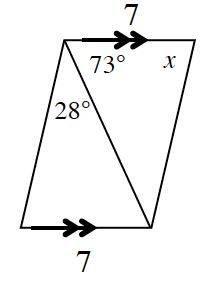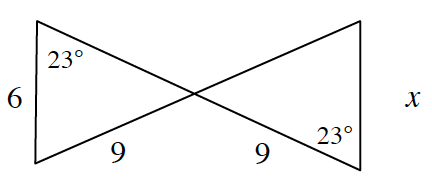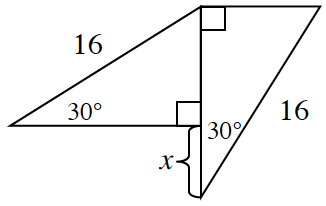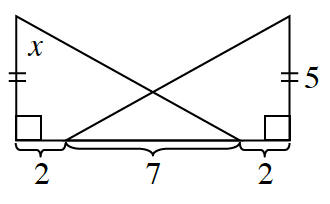### Home > CCG > Chapter 8 > Lesson 8.1.1 > Problem8-11

8-11.

Find the value of $x$ in each diagram below, if possible. If the triangles are congruent, state which triangle congruence property was used. If the triangles are not congruent or if there is not enough information, state, “Cannot be determined.”

Read the Math Notes box in Lesson 6.1.4 on the triangle congruence conjectures.
Are the two triangles in each part congruent?

1.To prove the triangles congruent notice both triangles have a side that is $7$.
Also, use alternate interior angles with the $73º$ angle.
Finally both triangles share the diagonal so based on the reflexive property these sides are congruent.
Finally the triangles are congruent by SAS congruence.
So using the triangle sum theorem, $x = 79º$.

1.Cannot be determined, because the triangles cannot be found to be congruent.
If the $23º$ angle was between the $6$ and the $9$ on both triangles
then the triangles would be congruent by ASA. Since they are not then the triangles are not congruent.

1.1.